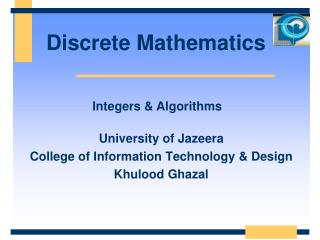DownloadDownload PresentationDiscrete Mathematics

# Discrete Mathematics

Télécharger la présentation## Discrete Mathematics

- - - - - - - - - - - - - - - - - - - - - - - - - - - E N D - - - - - - - - - - - - - - - - - - - - - - - - - - -
##### Presentation Transcript

1. Discrete Mathematics University of Jazeera College of Information Technology & Design Khulood Ghazal Integers & Algorithms

2. Euclidean Algorithm for GCD • Finding GCDs by comparing prime factorizations can be difficult if the prime factors are unknown. • Euclid discovered: For all integers a, b,gcd(a, b) = gcd((a mod b), b). • Sort a,b so that a>b, and then (given b>1) (a mod b) < a, so problem is simplified.

3. Euclidean Algorithm Example • gcd(372,164) = gcd(372 mod 164, 164). • 372 mod 164 = 372164372/164 = 372164·2 • = 372328 = 44. • gcd(164,44) = gcd(164 mod 44, 44). • 164 mod 44 = 16444164/44 = 16444·3 = 164132 = 32. • gcd(44,32) = gcd(44 mod 32, 32) = gcd(12, 32) = gcd(32 mod 12, 12) = gcd(8,12) = gcd(12 mod 8, 8) = gcd(4,8) = gcd(8 mod 4, 4) = gcd(0,4) = 4. • Note: See class notes for more Examples .

4. Euclid’s Algorithm Pseudocode procedure gcd(a, b: positive integers) whileb  0 r := amodb; a := b; b := r return a

5. Base-b number systems • Ordinarily we write base-10 representations of numbers (using digits 0-9). • 10 isn’t special; any base b>1 will work. • For any positive integers n, b, there is a unique sequence ak ak-1… a1a0of digitsai<b such that

6. Particular Bases of Interest Used only because we have 10 fingers • Base b=10 (decimal):10 digits: 0,1,2,3,4,5,6,7,8,9. • Base b=2 (binary):2 digits: 0,1. (“Bits”=“binary digits.”) • Base b=8 (octal):8 digits: 0,1,2,3,4,5,6,7. • Base b=16 (hexadecimal):16 digits: 0,1,2,3,4,5,6,7,8,9,A,B,C,D,E,F Usedinternally in all modern computers Octal digits correspond to groups of 3 bits Hex digits give groups of 4 bits

7. Converting to Base b (Algorithm, informally stated) • To convert any integer n to any base b>1: • To find the value of the rightmost (lowest-order) digit, simply compute n mod b. • Now replace n with the quotient n/b. • Repeat above two steps to find subsequent digits, until n is gone (=0). Note: See class notes for more Examples .

8. Addition of Binary Numbers procedureadd(an−1…a0, bn−1…b0: binary representations of non-negative integers a,b) carry := 0 forbitIndex := 0 to n−1 {go through bits} begin bitSum:=abitIndex+bbitIndex+carry{2-bit sum} sbitIndex := bitSummod 2 {low bit of sum} carry := bitSum/ 2 {high bit of sum} end sn := carry returnsn…s0: binary representation of integer s Note: See class notes for more Examples .

9. Two’s Complement • In binary, negative numbers can be conveniently represented using two’s complement notation. • In this scheme, a string of n bits can represent any integer i such that −2n−1 ≤ i < 2n−1. • The bit in the highest-order bit-position (n−1) represents a coefficient multiplying −2n−1; • The other positions i < n−1 just represent 2i, as before. • The negation of any n-bit two’s complement number a = an−1…a0 is given by an−1…a0 + 1.

10. Why two’s complement? binary 0111 0110 … 0001 0000 1111 1110 … 1001 1000 Decimal 7 6 … 1 0 -1 -2 … -7 -8 binary 1111 1110 … 0011 0010 0001 0000 Decimal 15 14 … 3 2 1 0

11. Why two’s complement? binary 0111 0110 … 0001 0000 1111 1110 … 1001 1000 Decimal 7 6 … 1 0 -1 -2 … -7 -8 binary 1111 1110 … 0011 0010 0001 0000 Decimal 15 14 … 3 2 1 0 Positive values 22,21,20 -23 Positive values 23,22,21,20

12. When you want to find the negative of a number, you take the number, and subtract it from zero No difference between subtracting the number from 0 and subtracting it from 2n to find the negative of an n-bit number, subtract the number from 0 or subtract it from 2n subtract our number from (1111 + 1) rather than 10000. (n=4 case)

13. Subtraction of Binary Numbers proceduresubtract(an−1…a0, bn−1…b0: binary two’s complement representations of integers a,b) returnadd(a, add(b,1)) { a + (−b) } This fails if either of the adds causes a carry into or out of the n−1 position, since 2n−2+2n−2 ≠ −2n−1, and −2n−1 + (−2n−1) = −2n isn’t representable! Note: See class notes for more Examples .

14. Multiplication of Binary Numbers proceduremultiply(an−1…a0, bn−1…b0: binary representations of a,bN) product := 0 fori := 0 to n−1 ifbi = 1 then product := add(an−1…a00i, product) returnproduct Note: See class notes for more Examples .